K-contact-flow

A contact form on a smooth-dimensional manifoldis a-formsuch that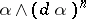is everywhere non-zero. The pair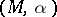is called a contact manifold. See also Contact structure.

A contact manifoldcarries a distinguished vector field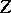, called the characteristic vector field or Reeb field, which is uniquely determined by the following equations:andfor all vector fields. The flow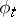generated by(when it is complete) is called the contact flow. Sometimes the name "contact flow" is used for the-dimensional foliation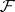consisting of the unparametrized orbits of, [a5].

If the flowis a Riemannian foliation in the sense of Reinhart–Molino [a7], i.e., if there is a holonomy-invariant transverse metric for, thenis called a-contact flow, and the pairis called a-contact manifold. This definition is equivalent to requiring that the flowofis a-parameter group of isometries for some contact metric (a Riemannian metricsuch that there exists an endomorphismof the tangent bundle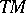such that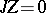,,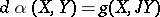, and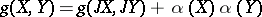for all vector fieldsandon). If one has in addition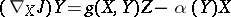, where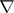is the Levi-Civita connection of, then one says thatis a Sasakian manifold, [a4], [a12].

As a consequence of the Meyer–Steenrod theorem [a6], a-contact flowon a compact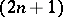-dimensional manifold is almost periodic: the closure ofin the isometry group of(of the associated contact metric) is a torus, of dimensionin betweenand, which acts onwhile preserving the contact form, [a3]. The "completely integrable" case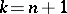has been studied in [a2]: these structures are determined by the image of their contact moment mapping.

The existence of-contact flows poses restrictions on the topology of the manifold. For instance, since a-contact flow can be approximated by a periodic-contact flow, only Seifert fibred compact manifolds can carry a-contact flow. Another example of a restriction is the Tachibana theorem, asserting that the first Betti number of a compact Sasakian manifold is either zero or even, [a9]. This shows that no torus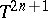can carry a Sasakian structure. Actually, P. Rukimbira [a8] showed that no torus can carry a-contact flow.

A. Weinstein [a11] has conjectured that the contact flow of a compact contact manifold has at least one periodic orbit. Despite important breakthroughs (including [a10]), this conjecture is not quite settled at present (1996). However, it is known that-contact flows on compact manifolds have at least two periodic orbits [a3].

Examples of-contact manifolds include the contact manifoldswith a periodic contact flow(these include the regular contact manifolds), such as the sphere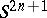equipped with the contact formthat is the restriction toof the-form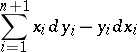on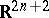. More generally, compact contact hypersurfaces (in the sense of M. Okumura) [a1] in Kähler manifolds of constant positive holomorphic sectional curvature carry-contact flows. A large set of examples is provided by the Brieskorn manifolds: In [a12] it is shown that every Brieskorn manifold admits many Sasakian structures, hence carries many-contact flows.